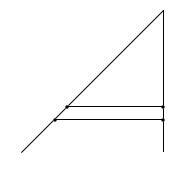# All line segments equal?

thinkandmull
Hello,

If someone takes two segments, one longer than the other, it is naturally assumed that the longer one is most definitely longer than the other. However, if the longer segment is put at an angle with the other to make a triangle, suddenly someone can draw a one to one correspondence between all the points on the original two segments. So the line was not really longer. How can we resolve this paradox?

## Answers and Replies

JonnyG
There is no paradox. Bijections do not, in general, preserve length.

Last edited:
Staff Emeritus
Homework Helper
I agree it looks weird and suspicious. But the correct resolution is to stop seeing it as a paradox and more as a fact of nature/math.

pixel
However, if the longer segment is put at an angle with the other to make a triangle, suddenly someone can draw a one to one correspondence between all the points on the original two segments.

Couldn't you do that even without putting the longer line at an angle with the other?

pixel
Is this what you have in mind?Here I'm showing two points on each line, with their distance exaggerated for clarity. Although there's a one to one correspondence, the distance between the two points on the longer line is greater than the distance between the two points on the shorter line.

Mentor
If someone takes two segments, one longer than the other, it is naturally assumed that the longer one is most definitely longer than the other.
The longer segment has a length that is larger, but both segments contain the same number of points. Another way to say this is that the cardinality of both segments is the same.